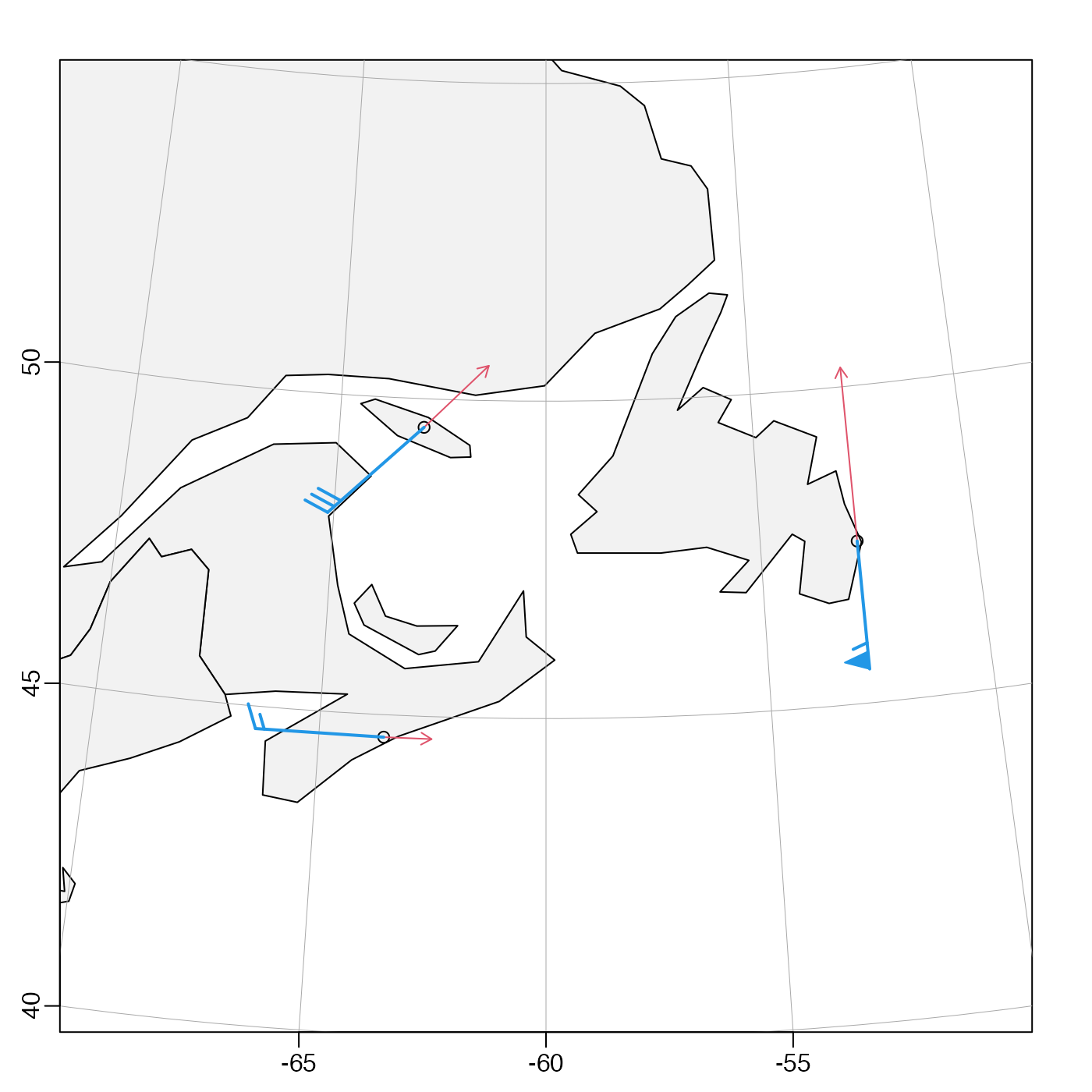Plot a direction field on a existing map.

mapDirectionField(
longitude,
latitude,
u,
v,
scale = 1,
length = 0.05,
code = 2,
col = par("fg"),
...
)

## Arguments

longitude, latitude numeric vectors of the starting points for arrows. numeric vectors of the components of a vector to be shown as a direction field. latitude degrees per unit of u or v. length of arrow heads, passed to arrows(). code of arrows, passed to arrows(). color of arrows. This may be a single color, or a matrix of colors of the same dimension as u. optional arguments passed to arrows(), e.g. angle and lwd can be useful in differentiating different fields.

## Details

Adds arrows for a direction field on an existing map. There are different possibilities for how longitude, latitude and u and v match up. In one common case, all four of these are matrices, e.g. output from a numerical model. In another, longitude and latitude are the coordinates along the matrices, and are thus stored in vectors with lengths that match appropriately.

A map must first have been created with mapPlot().

Other functions related to maps: formatPosition(), lonlat2map(), lonlat2utm(), map2lonlat(), mapArrows(), mapAxis(), mapContour(), mapCoordinateSystem(), mapGrid(), mapImage(), mapLines(), mapLocator(), mapLongitudeLatitudeXY(), mapPlot(), mapPoints(), mapPolygon(), mapScalebar(), mapText(), mapTissot(), oceCRS(), shiftLongitude(), usrLonLat(), utm2lonlat()

## Examples

# \donttest{
library(oce)
data(coastlineWorld)
par(mar=rep(2, 4))
mapPlot(coastlineWorld, longitudelim=c(-120,-55), latitudelim=c(35, 50),
proj="+proj=laea +lat0=40 +lat1=60 +lon_0=-110")lon <- seq(-120, -60, 15)
lat <- 45 + seq(-15, 15, 5)
lonm <- matrix(expand.grid(lon, lat)[, 1], nrow=length(lon))
latm <- matrix(expand.grid(lon, lat)[, 2], nrow=length(lon))
## vectors pointed 45 degrees clockwise from north
u <- matrix(1/sqrt(2), nrow=length(lon), ncol=length(lat))
v <- matrix(1/sqrt(2), nrow=length(lon), ncol=length(lat))
mapDirectionField(lon, lat, u, v, scale=3)mapDirectionField(lonm, latm, 0, 1, scale=3, col='red')#> Warning: zero-length arrow is of indeterminate angle and so skipped#> Warning: zero-length arrow is of indeterminate angle and so skipped#> Warning: zero-length arrow is of indeterminate angle and so skipped# Color code by longitude, using thick lines
col <- colormap(lonm)\$zcol
mapDirectionField(lonm, latm, 1, 0, scale=3, col=col, lwd=2)# }